Home > CCA2 > Chapter 10 > Lesson 10.1.4 > Problem10-70

10-70.
1.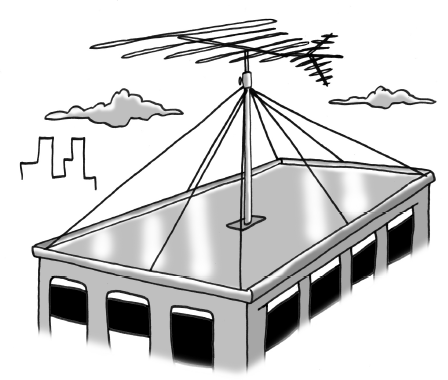The Flat Building’s roof is 32 feet wide and 60 feet long. An antenna rises 25 feet above the center of the roof, and wires connect the top of the antenna to each corner of the roof and to the midpoint of each edge, as shown in the picture at right. Homework Help ✎

1. What was the total length of wire used to connect the antenna to the roof (without counting any extra needed for attaching it)?

2. The wires that attach to the corners of the building form an angle with the roof. Find the measure of that angle.

3. Suppose the height of the antenna is x feet (instead of 25 feet). Represent the total length of the wires in terms of x.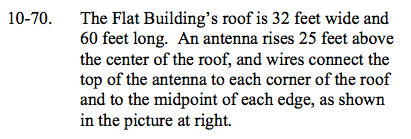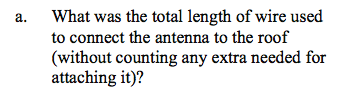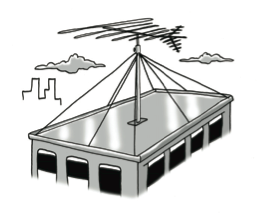First, find the length of the wire connected to the midpoint of the long edge.
You know that the antenna rises 25 feet (y) and the distance from the antenna to the edge is 16, half of the width (x).
Use the Pythagorean Theorem to find the length of the wire.

Use similar strategies to find the lengths of the remaining wires.
The total length is 306.26 feet of wire.

x2 + y2 = c2
162 + 252 = c2
c = 29.68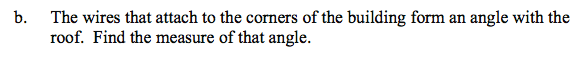Use inverse tangent to find the angle, using the lengths you derived from part (a).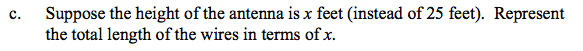Substitute x for the heights, and add the lengths of the wires.